## Friday, 28 October 2011

### Argand diagram VS cartesian coordinate

For those who lacks knowledge, the problems on complex numbers can be quite hard (Like those who're trying old Pure without some old ref book). That's why we need some simple transformation to make it equivalence to a 2D-co-geom problem.
Basic knowledge:
Complex number can be written in form of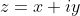where x and y are real numbers. Then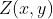represents the point z on the argand diagram.
The absolute value of the complex number: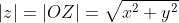The principal of complex number: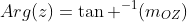where OZ is a vector such that angle beyond 180 degrees are considered.
Slope between two points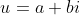and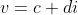:
Complex version: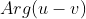Cartesian version: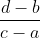Transform:
Complex version: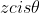Cartesian version: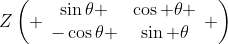Example 1: Let a, b be solutions of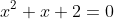while c and d be solutions of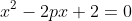. If the points of a,b,c and d on the argand diagram are concyclic, solve p.

Now consider the values of a,b: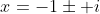And the values of c,d: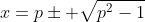Therefore c and d is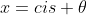or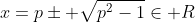When p>1, c=d and obviously ABCD isn't a quadrilateral, so p < 1.
Now transform it into geometrical problem: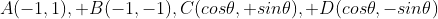are concyclic.
Note that:
1) ABCD is symmetrical about x-axis.
2) AB is parallel to x-axis.
We can conclude ABCD is a rectangle, therefore , p = 0.

Example 2: HKALE 1983 Q3
a) For complex number w, show that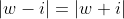if and only if w is real.
Standard ans:
If part: Let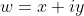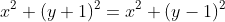,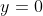.
Only if part: Let w be real. both sides trivially equal.

Co-geom analyse:
For circle, t's symmetrical along y-axis/Im axis. With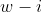being another point on the circle and the line joining w+i and w-i is perpendicular to the symmetrical axis, therefore the midpoint lies on the line joining center and perpendicular to the constructed line. i.e., w is on Re-axis, so w is real.

b) Sketch the locus of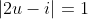I think complex number method would be faster here.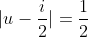, therefore the locus is a circle with center i/2 with radius 1/2.

Co-geom analysis: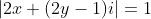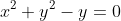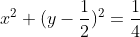, the same result is given.
c) Show thatis equivalent to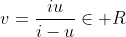, hence show that u,v and i are colinear.
The first part of question is easy when you "rationalize" v, you will have: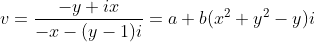, Im(v) =0 by our deduction before.

The second part is much more difficult, we should analyse the question in a geometrical manner:

Choose a point on, say U. The center of the circle C1 is X.
rotate the circle along the origin by 90 degress anticlockwisely to make the transform "iu" (Circle C2).

Now UX intersect the circle C1 again at U'. Assume iU intersect Re-axis at V'.
U' represents the point i-u. Note that OU is perpendicular to OU', i.e., the point U''(iu) lies on the line OU'. Therefore U', U'' and O are colinear.
Furthermore, by simple geometry, we can show that U'O is parallel to the line iU and iUOU' forms a rectangle. Then we can show that triangle U'OU and iOV' are in sprial rotation relationship. (similar triangle)

^ Actually we proved the first part of the question here.
Now for the second part of question:
By trigonometry means, and U'O^2 + UO^2 = 1, we can show that U''O:U'O = U'O : UO = 1: OV'. Therefore V lies on iU, Q.E.D.

Example 3: HKALE 1993 Q11
a) Show that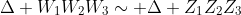iff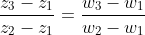.
b) Show that a triangle is equilateral iff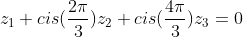.
c) Disprove the existance of equilateral triangle on argand diagram with all integral point vertices.

We will do the question seperately.

a) In geometrical approach, what can you see from the given information?
Information I: The quotient are equal. It implies that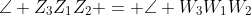.
Information II: The quotient are equal. It implies that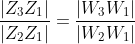.
Now apply the knowledge learnt in secondary 1. They are similar because angle equal, 2 sides proportional!!!!
We just get 4 mark easily from the AL Pure Maths paper XD

b) Consider another equilateral triangle with vertex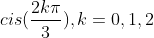.
Now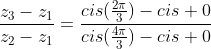, since cis 0 = 1,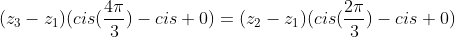By simple rearranging, we can obtain the above result.

c) Standard solution:
By equation in b, we have:
Equating real part: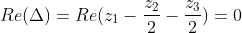Equating imaginary part: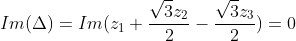Due to the closure of rational numbers under multiplication, we can show that the two equation does not have ALL rational solution. Hence there does not exist ALL integral solution.

Disclaimer: The above skills AREN'T answering skills, they are POSSIBLE solutions, but NOT PERFECT PRESENTATIONS. A RIGOROUS PROOF should be respected.

Exercise:
1) Consider example 3a:
a) Proof or disproof the existance of the general form proofing similar triangle on Argand diagram, showing the properties for i) AAA, ii) 3 sides proportional.
b) Write down one more requirement such that two triangles are congruent.
c) Suppose the two triangles are similar, write a general transformation between two triangles in terms of the complex numbers representing the points given.
d) Let triangle A,B,C,D,E be (1+i), (2+i), (3+3i), (4+5i), (-2+4i) respectively. If triangle ABC ~ triangle DEF where F is a point on the Argand diagram, find F and the transformation between two triangles.
2) Consider exmaple 3b:
a) Is the expression symmetrical for all three points of the triangle? Prove your assertion.
b) Equilateral triangle ABC has circumcircle |2u-i|=1. Write the general expression for the triangle.
c) Let A,B represent the point (1+i), (3+0i) respectively.
i) Find two points C and D such that ABC and ABD are equilateral.
ii) Show that CD is perpendicular to AB.
3) Consider example 2, in circle |2u-i|=1,
a) Show that (i-u), (0+i/2) and (u) are colinear.
b) Prove that (i,u), (u), (0) is an right-angled triangle.
c) Show that (iu) lies on line passing through (i-u) and 0.
4) Account the following FACT in terms of complex numbers:
a) Parallel lines do not intersect each other.
b) Angle bisectors of triangle are concurrent.
c) Ptolemy's theorem for both equality and inequality case. i.e. for quadrilateral ABCD,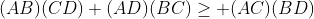, equality hold iff ABCD is concyclic.
5) HKALE 1984 Q6b: f(z) is defined by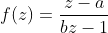are defined on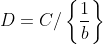where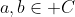. Suppose |f(1)|=|f(-1)|=|f(i)|=1.
a) Show that b is the conjugate of a, |f(z)| = 1 for all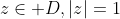.
b) Show that f(z) is a constant function if |a|=1.